Author: Oscar Cronquist Article last updated on October 09, 2017

According to wikipedia a round-robin tournament is a competition where all plays all. Excel is a great platform for building a round-robin tounament table and keeping scores.

You can use these custom functions below for creating a table for tennis, soccer, chess, bridge or whatever sport/competition schedule you want. At the very end of this post are instructions on how to use the custom functions. Let's start.

### Basic scheduling

The following vba code creates a schedule where each team plays once against another team.

```
Function roundrobin(rng As Range)
'Get Digital Help http://www.get-digital-help.com/
'Define variables
Dim tmp() As Variant, k As Long
Dim i As Long, j As Long

'ReDimension tmp variable
ReDim tmp(1 To (rng.Cells.Count / 2) * (rng.Cells.Count - 1), 1 To 2)
k = 1

'Schedule everyone with everyone
For i = 1 To rng.Cells.Count
For j = i + 1 To rng.Cells.Count
tmp(k, 1) = rng.Cells(i)
tmp(k, 2) = rng.Cells(j)
k = k + 1
Next j
Next i

'Return array
roundrobin = tmp

End Function
```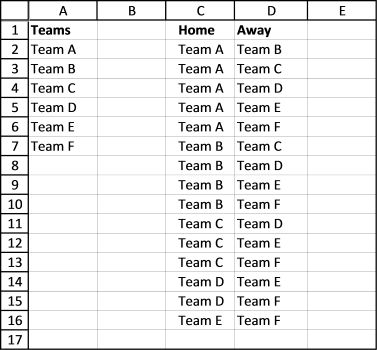As you can see it is not very complicated and the first team has home matches all the time. But if home and away doesn't matter this could useful.

Another bad thing with this custom function is that it doesn't split the schedule into rounds. A team can't play twice in the same round, obviously.

Also if you want the schedule to be somewhat random, this custom function is not for you.

The next custom function takes care of these three issues.

### Round-robin tournament

This custom function creates a round-robin tournament. It tries to distribute home and away rounds evenly and teams are randomly placed in the schedule.

```Function RoundRobin2(rng As Range)
'This custom function adds a team automatically if the number of teams is uneven.
'Get Digital Help http://www.get-digital-help.com/
Dim tmp() As Variant, k As Long, l As Integer
Dim i As Long, j As Long, a As Long, r As Long
Dim rngA As Variant, Stemp As Variant, Val As Long
Dim res, rngB() As Variant, result As String

'Transfer cell values to an array
rngA = rng.Value

'Check if the number of teams are even
If rng.Cells.Count Mod 2 = 0 Then
rngB = rng.Value
l = 0
Else
ReDim rngB(1 To UBound(rngA) + 1, 1 To 1)
For i = 1 To UBound(rngA)
rngB(i, 1) = rngA(i, 1)
Next i
rngB(UBound(rngB, 1), 1) = "-"
l = 1
End If

ReDim tmp(1 To ((rng.Cells.Count + l) / 2) * (rng.Cells.Count + l - 1), 1 To 3)

'Randomize array
rngB = RandomizeArray1(rngB)

Val = (UBound(rngB, 1) / 2)
'Build schedule
For i = 2 To UBound(rngB, 1)

a = 1
For r = 1 To (UBound(rngB, 1) / 2)
tmp(r + Val * (i - 2), 1) = i - 1
If (i - 1) Mod 2 = 1 Then
tmp(r + Val * (i - 2), 2) = rngB(a, 1)
tmp(r + Val * (i - 2), 3) = rngB(UBound(rngB, 1) - a + 1, 1)
Else
tmp(r + Val * (i - 2), 2) = rngB(UBound(rngB, 1) - a + 1, 1)
tmp(r + Val * (i - 2), 3) = rngB(a, 1)
End If
a = a + 1
Next r

'switch places for all values except the first one
For j = 2 To UBound(rngB, 1) - 1
Stemp = rngB(j, 1)
rngB(j, 1) = rngB(j + 1, 1)
rngB(j + 1, 1) = Stemp
Next j
Next i

RoundRobin2 = tmp

End Function
```

This user defined function creates a random schedule split into rounds, home and away are also somewhat evenly distributed through the schedule.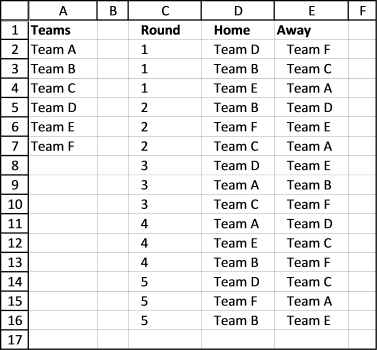This table shows you how many times 6 teams play home and away for the entire tournament.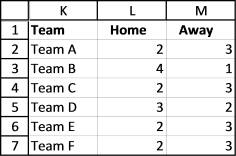### Double round-robin tournament

To make sure every team has as many home as away rounds competitors play each other twice. It is a "double" round-robin tournament. The way it works is all play all twice, once home and once away.

```Function doubleroundrobin(rng As Range)
'Get Digital Help http://www.get-digital-help.com/
Dim tmp() As Variant, k As Long, l As Integer
Dim i As Long, j As Long, a As Long, r As Long
Dim rngA As Variant, Stemp As Variant, Val As Long
Dim res, rngB() As Variant, result As String, cc As Long

'Transfer values to an array
rngA = rng.Value

'Check if the number of teams are even
If rng.Cells.Count Mod 2 = 0 Then
rngB = rng.Value
l = 0
Else
ReDim rngB(1 To UBound(rngA) + 1, 1 To 1)
For i = 1 To UBound(rngA)
rngB(i, 1) = rngA(i, 1)
Next i
rngB(UBound(rngB, 1), 1) = "-"
l = 1
End If

cc = ((rng.Cells.Count + l) / 2) * (rng.Cells.Count + l - 1)

ReDim tmp(1 To cc * 2, 1 To 3)

'Randomize array
rngB = RandomizeArray1(rngB)

Val = (UBound(rngB, 1) / 2)

'Build schedule
For i = 2 To UBound(rngB, 1)

a = 1
For r = 1 To (UBound(rngB, 1) / 2)
tmp(r + Val * (i - 2), 1) = i - 1
If (i - 1) Mod 2 = 1 Then
tmp(r + Val * (i - 2), 2) = rngB(a, 1)
tmp(r + Val * (i - 2), 3) = rngB(UBound(rngB, 1) - a + 1, 1)
Else
tmp(r + Val * (i - 2), 2) = rngB(UBound(rngB, 1) - a + 1, 1)
tmp(r + Val * (i - 2), 3) = rngB(a, 1)
End If
a = a + 1
Next r
For j = 2 To UBound(rngB, 1) - 1
Stemp = rngB(j, 1)
rngB(j, 1) = rngB(j + 1, 1)
rngB(j + 1, 1) = Stemp
Next j
Next i

'Copy schedule and change home to away and vice versa, this makes it a double round-robin tournament
For i = cc + 1 To cc * 2
tmp(i, 1) = UBound(rngB, 1) - 1 + tmp(i - cc, 1)
tmp(i, 2) = tmp(i - cc, 3)
tmp(i, 3) = tmp(i - cc, 2)
Next i

doubleroundrobin = tmp

End Function
```Here is a table that shows you teams play as many home as away games.

### Macro

The macros in the workbook below allows you to create a match schedule. Go to sheet Macro and follow instructions.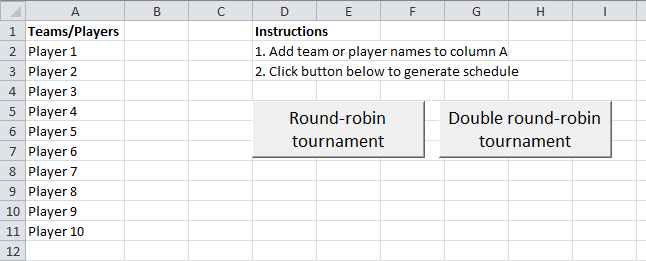Add teams or players to column A, then click "Round-robin tournament" button or "Double round-robin tournament".

A match schedule is created on a new sheet.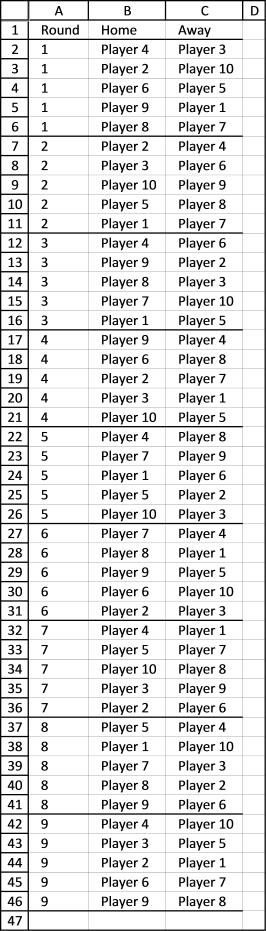Conditional formatting separates rounds with a line, it makes the table easier to read.

Round-robin tournament

```Sub rr()
Dim Lrow As Long
Dim rng As Range, tmp() As Variant
Dim ws As Worksheet
Application.ScreenUpdating = False
Lrow = Worksheets("Macro").Range("A" &amp; Rows.Count).End(xlUp).Row
Set rng = Worksheets("Macro").Range("A2:A" &amp; Lrow)

tmp = RoundRobin2(rng)

'Insert new sheet

ws.Range("A1") = "Round"
ws.Range("B1") = "Home"
ws.Range("C1") = "Away"
ws.Range("A2").Resize(UBound(tmp, 1), 3) = tmp

ws.Range("A1").Resize(UBound(tmp, 1) + 1, 3).InsertIndent 1
Columns("A:C").EntireColumn.AutoFit
ws.Range("A1:C1000").Select
Selection.FormatConditions(Selection.FormatConditions.Count).SetFirstPriority
With Selection.FormatConditions(1).Borders(xlBottom)
.LineStyle = xlContinuous
.Weight = xlThin
End With
Selection.FormatConditions(1).StopIfTrue = False
Application.ScreenUpdating = True
End Sub
```

Double round-robin tournament

```Sub droundrobin()
Dim Lrow As Long
Dim rng As Range, tmp() As Variant
Dim ws As Worksheet
Application.ScreenUpdating = False
Lrow = Worksheets("Macro").Range("A" &amp; Rows.Count).End(xlUp).Row
Set rng = Worksheets("Macro").Range("A2:A" &amp; Lrow)

tmp = doubleroundrobin(rng)

'Insert new sheet

ws.Range("A1") = "Round"
ws.Range("B1") = "Home"
ws.Range("C1") = "Away"
ws.Range("A2").Resize(UBound(tmp, 1), 3) = tmp

ws.Range("A1").Resize(UBound(tmp, 1) + 1, 3).InsertIndent 1
Columns("A:C").EntireColumn.AutoFit
ws.Range("A1:C1000").Select
Selection.FormatConditions(Selection.FormatConditions.Count).SetFirstPriority
With Selection.FormatConditions(1).Borders(xlBottom)
.LineStyle = xlContinuous
.Weight = xlThin
End With
Selection.FormatConditions(1).StopIfTrue = False
Application.ScreenUpdating = True
End Sub
```

This function moves values in an array randomly.

```Function RandomizeArray1(Arr As Variant)
Dim temp
Dim i As Long, j As Long, k As Long
Dim result As Variant

For k = LBound(Arr, 1) To UBound(Arr, 1)
result = result &amp; Arr(k, 1) &amp; " "
Next k
result = result &amp; vbNewLine

For i = LBound(Arr, 1) To UBound(Arr, 1)

j = Application.WorksheetFunction.RandBetween(LBound(Arr, 1), UBound(Arr, 1))
temp = Arr(j, 1)
Arr(j, 1) = Arr(i, 1)
Arr(i, 1) = temp
For k = LBound(Arr, 1) To UBound(Arr, 1)
result = result &amp; Arr(k, 1) &amp; " "
Next k
result = ""
Next i

RandomizeArray1 = Arr

End Function
```

round-robin-tournament.xlsm

### How to use a custom function

If you want the vba code in your own workbook, do this.

1. Press Alt-F11 to open visual basic editor
2. Click Module on the Insert menu
3. Copy and paste all custom functions above to the code module4. Exit visual basic editor (Alt+Q)
5. Save your workbook as an *.xlsm file

Now you can use the custom functions. Type your teams in a column. Select a blank cell range, 3 columns wide and many rows, you can extend this later if not all rounds show up.

Type =doubleroundrobin(cell_ref_to_your_teams), press and hold CTRL + Shift. Press Enter. Release all keys.